Learning Library

# Fraction Practice

The fraction practice offered in this set of worksheets will help build a strong foundation in math skills. Kids will color in fractions, add them, and subtract them, as well as decide which fractions are greatest, least or equivalent. Understanding fractions is an essential math skill for third graders to master, and when it comes to math, practice makes perfect.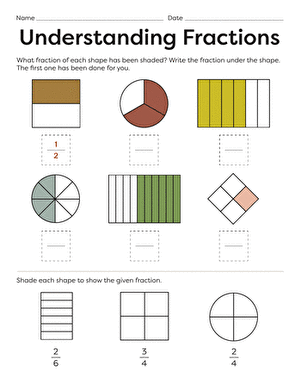### Find the Fraction

Each shape on this third grade math worksheet is divided into equal parts, and some of the parts are shaded. Kids identify and write the fraction represented.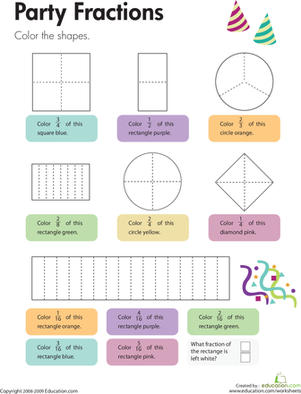### "Color-fill" Fractions

Each geometric shape on this third grade math worksheet is divided into equal parts. Kids color each shape to represent a certain fraction.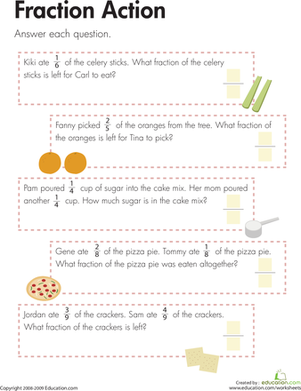### Word Up! Fraction Word Problems

Eating fractions is a great way to advance kids' understanding of fractions. This worksheet asks kids to solve fraction word problems about food.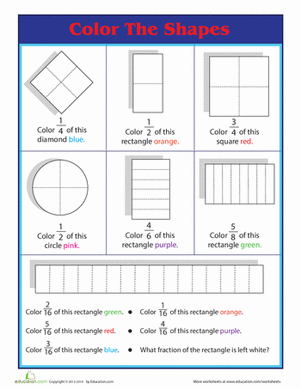### Portion Control: Color the Fractions

Hunting for a worksheet to help with fractions? This printable uses geometry to help your child get a handle on fractions.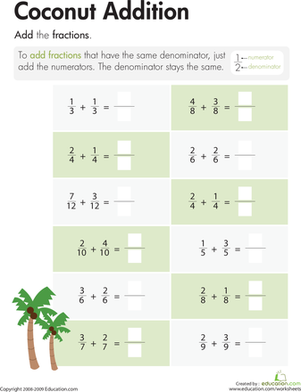### Crazy Coconut Fractions

On this third grade math worksheet, kids add simple fractions that have the same denominator.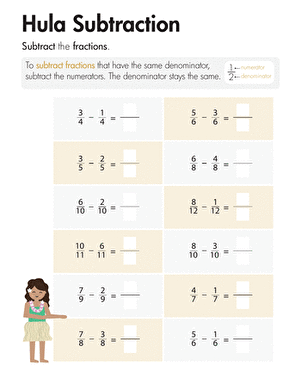### Hula Subtraction

On this third grade math worksheet, kids subtract simple fractions that have the same denominator.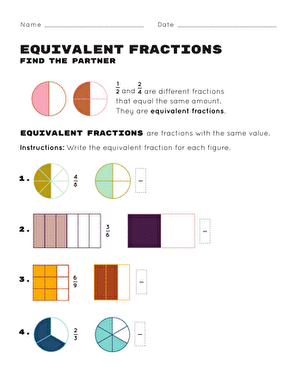### Equivalent Fractions: Find the Partner

This equivalent fractions worksheet helps your child learn about and create equivalent fractions. Try this equivalent fractions worksheet with your child.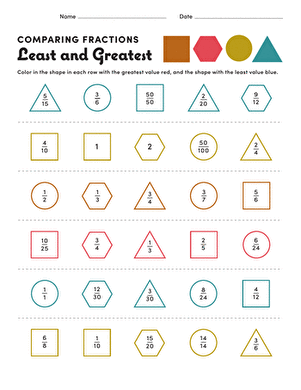### Comparing Fractions: Least and Greatest

Here's a math challenge for your 4th grader: in this worksheet, your child will determine which fraction is greatest and which is least.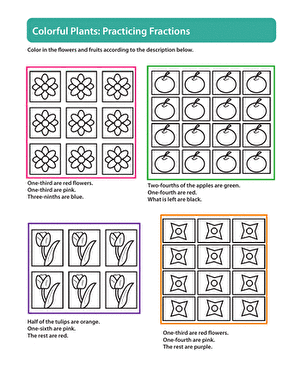### Gardening with Fractions

In this 4th grade worksheet, your child will practice reducing fractions as she colors each plot of plants according to the directions given.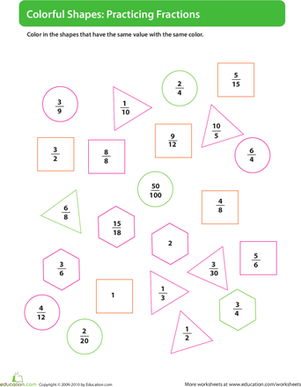### Color by Fraction

Here's a challenging math worksheet for your 4th grader: your child must color the fractions according to their value, giving each value its own color.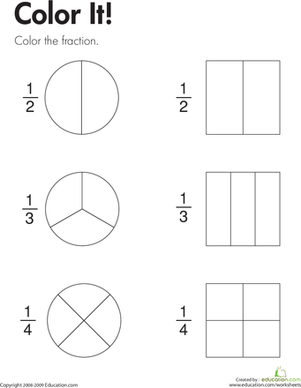### Fraction Practice: Color It!

To complete this second grade math worksheet, kids color parts of shapes to show the fractions 1/2, 1/3, and 1/4.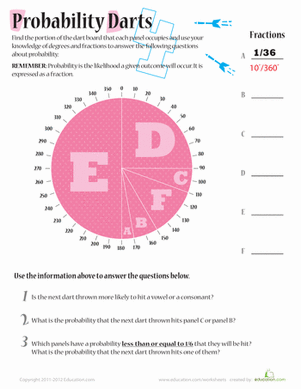### Probability Darts 4

Building on math skills with fractions, addition, and degrees, kids will figure out how much of the dart board each panel takes up then answer questions.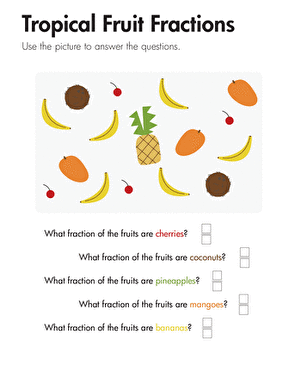### Tropical Fruit Fractions

This third grade math worksheet asks questions about a set of different kinds of fruit. Kids respond to the questions by counting to determine the fractions.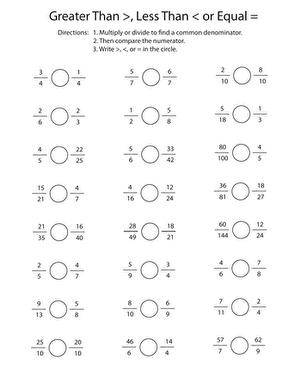### Greater Than or Less Than? Comparing Fractions

Covering a variety of fraction skills such as converting to like fractions, this worksheet is sure to challenge your fifth grader's fraction savvy.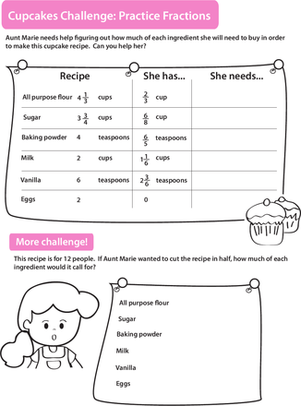### Baking with Fractions

In this 5th grade math worksheet, your child will work with fractions as she calculates how much of each ingredient she needs for a recipe.

Create new collection

0

### New Collection>

0 items

What could we do to improve Education.com?